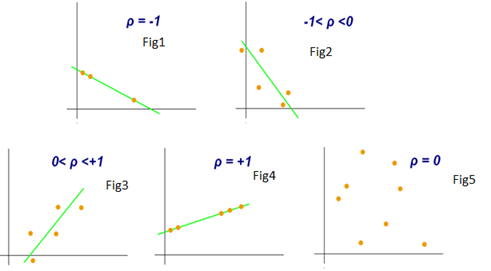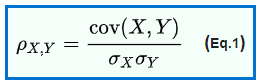# Pearson correlation coefficientAs Covariance has limitation to quantify the relationship, there is another concept called Pearson correlation coefficient (PCC) that overcome this limitation.

In my previous blog, we learnt about Covariance to measure relationship between two random variables.

### Covariance

In this blog, we’ll try to understand how to measure relationships between random variables.

medium.com

As Covariance has limitation to quantify the relationship, there is another concept called Pearson correlation coefficient (PCC) that overcome this limitation. It’s often represented with the Greek alphabet ρ. So the Pearson correlation coefficient between two random variables x and y is nothing but the covariance( X, Y) divided by the standard deviation of x and the standard deviation of y. Here is the mathematical formula for ρ.Now, you might ask, why are we defining a new metric? Because covariance doesn’t take variability in account, and here we use the standard deviation of x and y in denominator.

What exactly standard deviation of x is? It is nothing but square root of variance of x, and variance is all about variability.

When you measure covariance, you’re not measuring the variability within x&y. But just a small modification on covariance i.e (dividing your covariance by a standard deviation of x and standard deviation of y) will give you variability and interpretability.

As we saw in last blog on Covariance i.e, as x increases, if y also increase, then covariance is going to be positive. But how much positive? It could be very, very positive or very negative, right? Similarly, I know that as x increases, y decreases, my covariance is going to be negative. Right? But I don’t know how much negative..

So PCC is a very nice idea to quantify the relationship, Below graph gives a better understanding on PCC.

## Statistical Tests for Data Analysis Part-I

These statistical tests allow researchers to make inferences because they can show whether an observed pattern is due to intervention or chance. There is a wide range of statistical tests.

## Variables — What are they?

Variable is a quantity that may vary from object to object. For example, we measure heights of 50 mango trees in a selected plot and

## Global Terrorism Study : Exploratory and Descriptive Data Analysis

Global Terrorism Database Analysis was a quick project for understanding and implementing various descriptive statistics and exploratory data analysis techniques.

## Exploratory Data Analysis is a significant part of Data Science

Data science is omnipresent to advanced statistical and machine learning methods. For whatever length of time that there is data to analyse, the need to investigate is obvious.

## Permissible transforms and permissible statistics

Permissible statistics define a set of statistical tests that are allowed at each level of measurements. While tests allowed at higher levels cannot be performed at lower levels, vice-versa is acceptable.